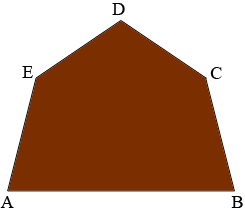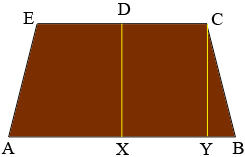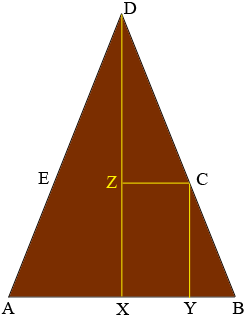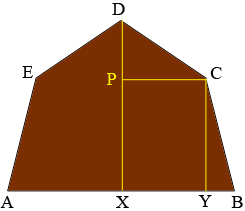SEARCH HOMEMath Central Quandaries & QueriesQuestion from Joe: Is it possible to build a barn roof (irregular pentagon?) with a 12' base and the other 4 sides 4' each? Thanks.Hi Joe,

I assume this is the shape you want.If so then the answer is yes but you don't have a lot of choice.

If the roof is as short as possible then $CDE$ is a straight line and the distance from $X$ to $Y$ is 4 feet.If the roof is as tall as possible then $BCD$ is a straight line and $|BC|=|CD|,$ although hey don't look equal in my diagram. Thus triangles $YBC$ and $ZCD$ are congruent and hence $|XY| = |ZC|= |YB|$ and thus the distance from $X$ to $Y$ is 3 feet.Hence choose a point Y on the base between 3 and 4 feet from the center point $X.$ Since the triangle $YBC$ is a right triangle the height $|YC|$ can then be found using Pythagoras theorem.

$|BC|^2 = |YB|^2 + |YC|^2$

so

$|YC| = \sqrt{4^2 - |YB|^2}.$In a similar fashion

$|PD| = \sqrt{4^2 - |PC|^2}.$

I hope this helps,
PennyMath Central is supported by the University of Regina and the Imperial Oil Foundation.